# Lesson 38 Implicit Differentiation Calculus Santowski 6152021 Calculus

• Slides: 15Lesson 38 – Implicit Differentiation Calculus - Santowski 6/15/2021 Calculus - Santowski 1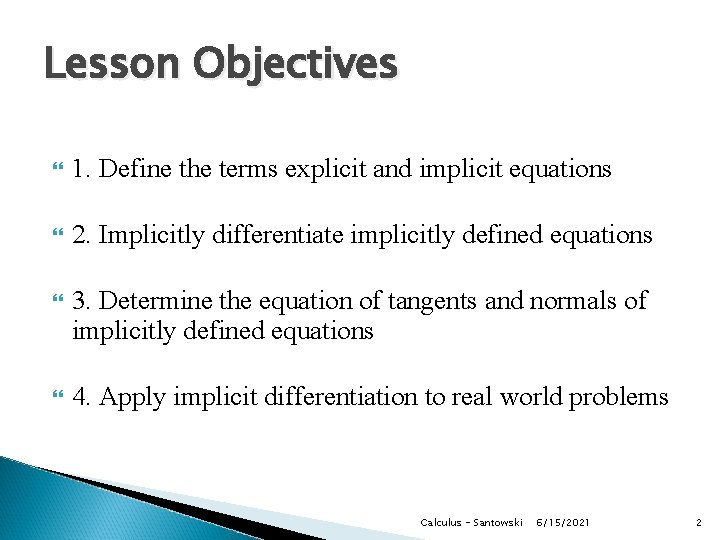Lesson Objectives 1. Define the terms explicit and implicit equations 2. Implicitly differentiate implicitly defined equations 3. Determine the equation of tangents and normals of implicitly defined equations 4. Apply implicit differentiation to real world problems Calculus - Santowski 6/15/2021 2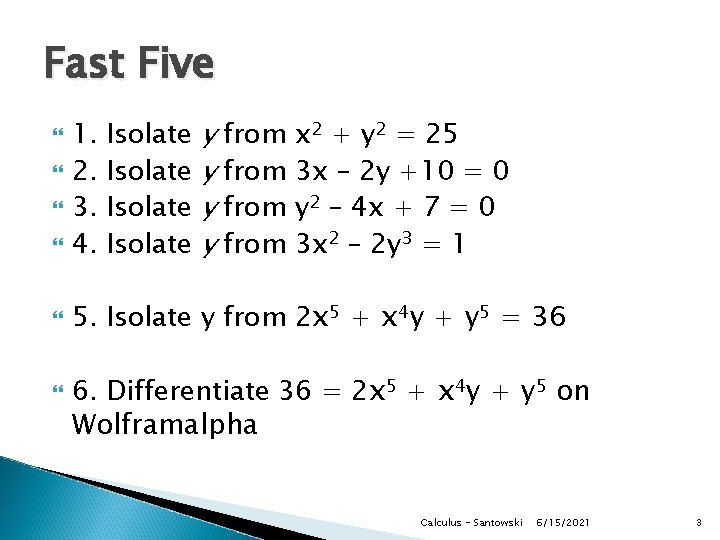Fast Five 5. Isolate y from 2 x 5 + x 4 y + y 5 = 36 Isolate y from x 2 + y 2 = 25 y from 3 x – 2 y +10 = 0 y from y 2 – 4 x + 7 = 0 y from 3 x 2 – 2 y 3 = 1 1. 2. 3. 4. 6. Differentiate 36 = 2 x 5 + x 4 y + y 5 on Wolframalpha Calculus - Santowski 6/15/2021 3(A) Review Up to this point, we have always defined functions by expressing one variable explicitly in terms of another i. e. y = f(x) = x 2 - 1/x + x In other courses, we have also seen functions expressed implicitly i. e. in terms of both variables i. e. x 2 + y 2 = 25 In simple implicit functions, we can always isolate the y term to rewrite the equation in explicit terms ◦ i. e. y = + (25 – x 2) In other cases, rewriting an implicit relation is not so easy i. e. 2 x 5 + x 4 y + y 5 = 36 Calculus - Santowski 6/15/2021 4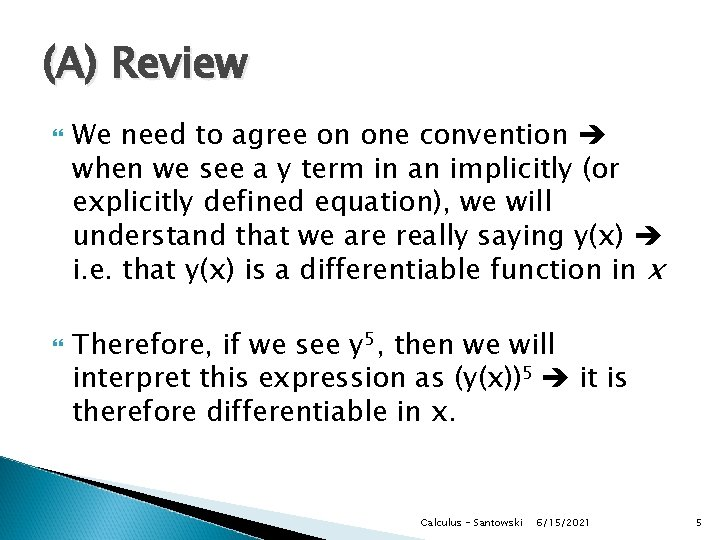(A) Review We need to agree on one convention when we see a y term in an implicitly (or explicitly defined equation), we will understand that we are really saying y(x) i. e. that y(x) is a differentiable function in x Therefore, if we see y 5, then we will interpret this expression as (y(x))5 it is therefore differentiable in x. Calculus - Santowski 6/15/2021 5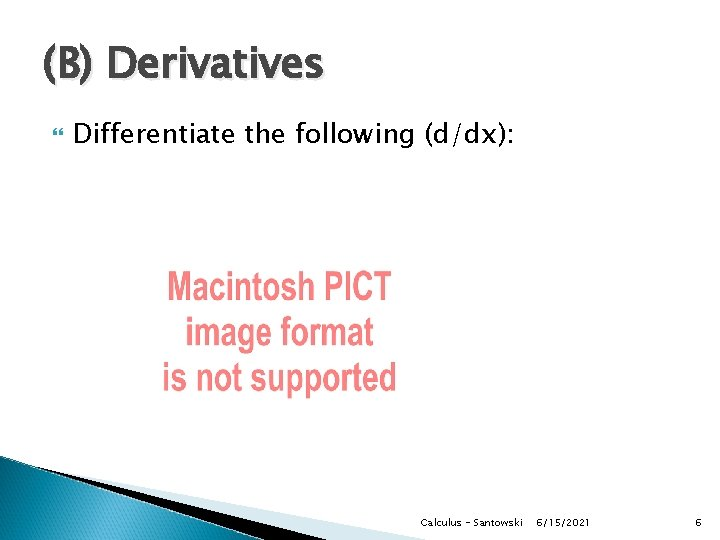(B) Derivatives Differentiate the following (d/dx): Calculus - Santowski 6/15/2021 6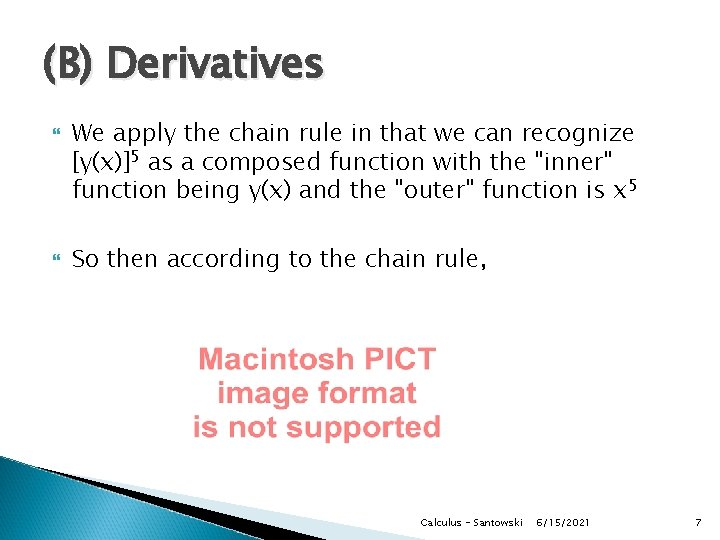(B) Derivatives We apply the chain rule in that we can recognize [y(x)]5 as a composed function with the "inner" function being y(x) and the "outer" function is x 5 So then according to the chain rule, Calculus - Santowski 6/15/2021 7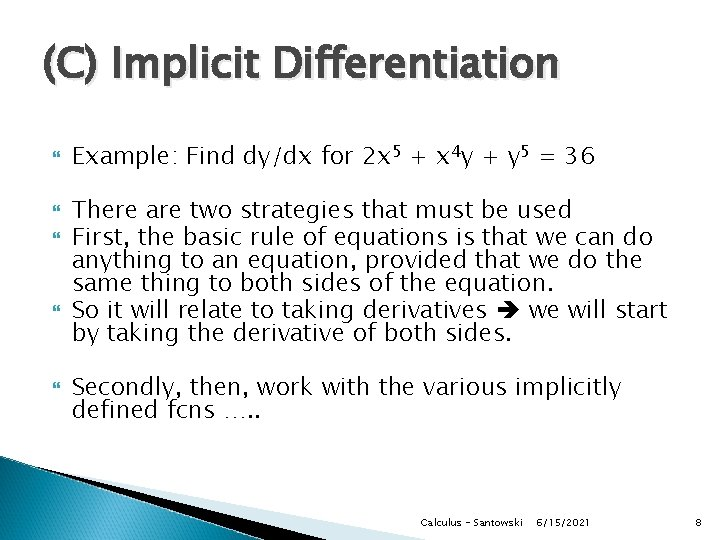(C) Implicit Differentiation Example: Find dy/dx for 2 x 5 + x 4 y + y 5 = 36 There are two strategies that must be used First, the basic rule of equations is that we can do anything to an equation, provided that we do the same thing to both sides of the equation. So it will relate to taking derivatives we will start by taking the derivative of both sides. Secondly, then, work with the various implicitly defined fcns …. . Calculus - Santowski 6/15/2021 8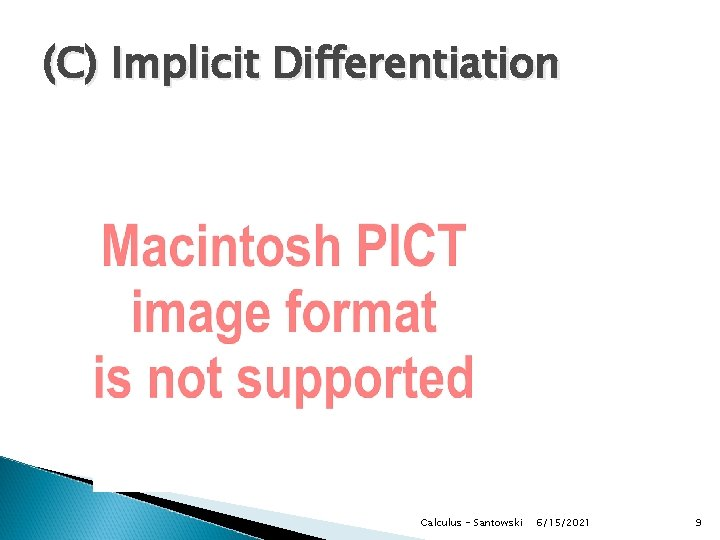(C) Implicit Differentiation Calculus - Santowski 6/15/2021 9(D) In Class Examples ex 2. Find dy/dx if x + y = x 2 y 3 + 5 ex 3. Find the slope of the tangent line drawn to x 2 + 2 xy + 3 y 2 = 27 at x = 0. ex 4. Determine the equation of the tangent line to the ellipse 4 x 2 + y 2 - 8 x + 6 y = 12 at x = 3. ex 5. Find d 2 y/dx 2 of x 3 + y 3 = 6 xy Calculus - Santowski 6/15/2021 10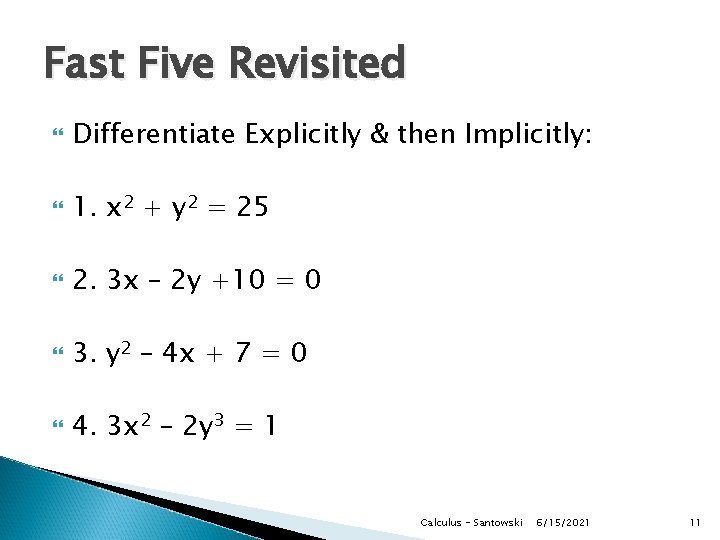Fast Five Revisited Differentiate Explicitly & then Implicitly: 1. x 2 + y 2 = 25 2. 3 x – 2 y +10 = 0 3. y 2 – 4 x + 7 = 0 4. 3 x 2 – 2 y 3 = 1 Calculus - Santowski 6/15/2021 11(D) In Class Example Differentiate the following: Calculus - Santowski 6/15/2021 12“Level 7” Level Questions 1. Find the equation of the lines that are tangent to the ellipse x 2 + 4 y 2 = 16 AND also pass through the point (4, 6) 2. Prove that the curves defined by x 2 – y 2 = k and xy = p intersect orthogonally for all values of of the constants k and p. Illustrate with a sketch Calculus - Santowski 6/15/2021 13“Level 7” Level Questions Find the equation of the tangent line at the point (a, b) on the curve x 2/3 + y 2/3 = 1. Hence, show that the distance between the xand y-intercepts of the tangent line is independent of the point of tangency Calculus - Santowski 6/15/2021 14Resources You can watch the following ppt/pdfs: http: //mrsantowski. tripod. com/2014 Math. HL/Resources/Impl icit_Diff_Part_1. pdf http: //mrsantowski. tripod. com/2014 Math. HL/Resources/Impl icit_Diff_Part_2. pdf https: //www. youtube. com/watch? v=anq_8 ARu 08 g https: //www. youtube. com/watch? v=rt. Zqp. BSow. JU https: //www. youtube. com/watch? v=1 sc. Xr 6 g 7 Hd. A Calculus - Santowski 6/15/2021 15